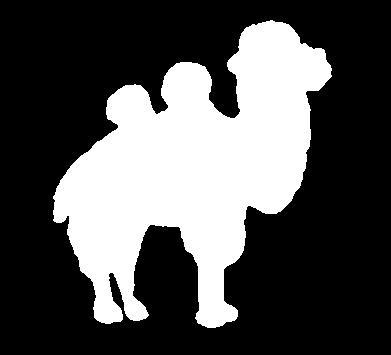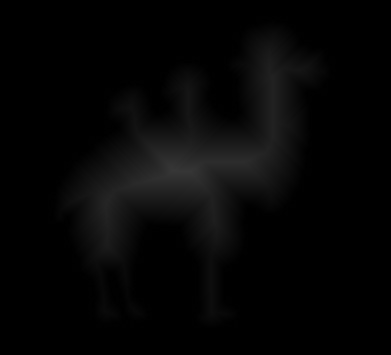2017-04-20 22:05:05 chenhuiian 阅读数 970
• ###### 征服C++ 11视频精讲

扫码进入学习群，领取学习资料+讲师答疑 本教程是C++ Primer Plus 6th 、The C++ Programming Language_4th、Thinking in C++等国外顶级的C++著作的精华和个人超过10年的C++使用经验的完美结合。

78928 人正在学习 去看看 李宁

**c++实现sobe**l

sobel 是一种边缘检测算子，有x和y方向的3*3的模板。sobel原理还是很简单的，但是写代码的时候要注意几个细节问题。

``````#include<iostream>
#include<opencv2/highgui/highgui.hpp>
#include<opencv2/imgproc/imgproc.hpp>
using namespace cv;
using namespace std;

int xGradient(Mat img, int x, int y);
int yGradient(Mat img, int x, int y);

int main()
{
int Gx, Gy, sum;
Mat dst = img.clone();
if(!img.data)
return -1;

for ( int y = 0; y < img.rows; y++)
{
for( int x = 0; x < img.cols; x++)
{
dst.at<uchar>(y,x) = 0.0;
}
}

for ( int y = 1; y < img.rows - 1; y++ )
{
for ( int x = 1; x < img.cols - 1 ; x++ )//错误原因，-1忘了，所以维数不匹配
{
sum = abs(Gx) + abs(Gy);
sum = sum > 255 ? 255 : sum;
sum = sum < 0 ? 0 : sum;
dst.at<uchar>(y,x) = sum;
}
}

imshow("final",dst);
waitKey(0);
return 0;

}

int xGradient(Mat image, int x, int y)
{
int gx;
gx = image.at<uchar>(y-1,x+1) + 2*image.at<uchar>(y,x+1) + image.at<uchar>(y+1,x+1)
- image.at<uchar>(y-1,x-1) - 2*image.at<uchar>(y,x-1) -image.at<uchar>(y-1,x-1);
return gx;
}
int yGradient(Mat image, int x, int y)
{
int gy;
gy = image.at<uchar>(y-1,x+1) + 2*image.at<uchar>(y-1,x) + image.at<uchar>(y+1,x+1)
- image.at<uchar>(y-1,x-1) - 2*image.at<uchar>(y+1,x) -image.at<uchar>(y-1,x-1);
return gy;
}``````

1. 在opencv里，图像的坐标系是以左上角为原点，水平向右为x轴，竖直向下为y轴，也就是说img.rows其实是Y轴方向，img.cols是x轴方向。在使用img.at(y,x)应该这样表达。
2. 在进行卷积的时候，要记得将边缘像素空出，不然会出现维数不合的错误。

sobel的算法思路还是很简单的，要注意的就是细致！

2017-06-21 21:46:28 wfei101 阅读数 5025
• ###### 征服C++ 11视频精讲

扫码进入学习群，领取学习资料+讲师答疑 本教程是C++ Primer Plus 6th 、The C++ Programming Language_4th、Thinking in C++等国外顶级的C++著作的精华和个人超过10年的C++使用经验的完美结合。

78928 人正在学习 去看看 李宁计算旋转后图像时就可以利用(3)式用旋转后的坐标计算其在原图中的坐标，并利用附近的像素插值的到当前的像素值。

图像的原点一般在左上角，这样计算出来的坐标会有负值，一般将图像原点平移到图像中心。记原图像的宽高为srcW、srcH，旋转后图像宽高为dstW、dstH，那么(3)式就变为：由旋转后图像坐标计算出来的原图像坐标往往不是整数，为了保证旋转效果，进行双线性插值。双线性插值就是对两个变量的线性插值，分别对每个变量线性插值就得到最终的插值结果。[cpp] view plain
1. void main(int argc, char** argv)
2. {
4.     unsigned char* pColorImg=NULL;
5.     int width=iplOrg->width;
6.     int height=iplOrg->height;
7.     pColorImg=(unsigned char*)malloc(width*height*3*sizeof(unsigned char));
8.
9.     cvCvtColor(iplOrg,iplOrg,CV_BGR2RGB);
10.     IplToUchar(iplOrg,pColorImg);   //用数组表示图像
11.     cvReleaseImage(&iplOrg);
12.
13.     double degree=15;   //逆时针旋转角度0~180
14.
15.     int tempLength=sqrt((double)width * width + (double)height *height) + 10;//保证原图可以任意角度旋转的最小尺寸
16.     unsigned char* pTemp=(unsigned char*)malloc(tempLength*tempLength*3*sizeof(unsigned char));
17.
18.
19.     //旋转
20.     myImgRotate(pColorImg,width,height,pTemp,tempLength,tempLength,degree,3);
21.     DisplayPicture(tempLength,tempLength,pTemp,"rotate.bmp",3); //保存图像
22.
23.     free(pTemp);
24.     pTemp=NULL;
25.     free(pColorImg);
26.     pColorImg=NULL;
27.
28. }

[cpp] view plain
1. //逆时针旋转到pdst的中心，其它用0填充
2. //pSrc,srcW,srcH原图及其尺寸
3. //pDst,dstW,dstH旋转后图像及其尺寸
4. //旋转角度
5. //通道数
6. void myImgRotate(unsigned char* pSrc,int srcW,int srcH,
7.                            unsigned char* pDst,int dstW,int dstH,
8.                            double degree,int nchannel)
9. {
10.
11.     int k;
12.     double angle = degree  * 3.1415926 / 180.;  //旋转角度
13.     double co=cos(angle);   //余弦
14.     double si=sin(angle);   //正弦
15.     int rotateW,rotateH;    //旋转后图像的高宽
16.     int srcWidthStep=srcW*nchannel;//宽度步长
17.     int dstWisthStep=dstW*nchannel;
18.     int x,y;
19.     int xMin,xMax,yMin,yMax;
20.     int xOff,yOff;  //偏移
21.     double xSrc=0.;
22.     double ySrc=0.; //变换后图像的坐标在原图中的坐标
23.
24.     //临时变量
25.     float valueTemp=0.;
26.     float a1,a2,a3,a4;
27.
28.     memset(pDst,0,dstWisthStep*dstH*sizeof(unsigned char));
29.     //计算旋转后的坐标范围
30.     rotateH=srcW*fabs(si)+srcH*fabs(co);
31.     rotateW=srcW*fabs(co)+srcH*fabs(si);
32.
33.     //计算偏移
34.     xOff=dstW/2;
35.     yOff=dstH/2;
36.
37.     yMin=(dstH-rotateH)/2.;
38.     yMax=yMin+rotateH+1;    //加1
39.     xMin=(dstW-rotateW)/2.;
40.     xMax=xMin+rotateW+1;
41.
42.     for (y=yMin;y<=yMax;y++)
43.     {
44.         for (x=xMin;x<=xMax;x++)
45.         {
46.             //求取在原图中的坐标
47.             ySrc=si*double(x-xOff)+co*double(y-yOff)+double(int(srcH/2));
48.             xSrc=co*double(x-xOff)-si*double(y-yOff)+double(int(srcW/2));
49.
50.             //如果在原图范围内
51.             if (ySrc>=0. && ySrc<srcH-0.5 && xSrc>=0. && xSrc<srcW-0.5)
52.             {
53.                 //插值
54.                 int xSmall=floor(xSrc);
55.                 int xBig=ceil(xSrc);
56.                 int ySmall=floor(ySrc);
57.                 int yBig=ceil(ySrc);
58.
59.                 for (k=0;k<nchannel;k++)
60.                 {
61.                     a1=(xSmall>=0 && ySmall>=0 ? pSrc[ySmall*srcWidthStep+xSmall*nchannel+k]:0);
62.                     a2=(xBig<srcW && ySmall>=0 ? pSrc[ySmall*srcWidthStep+xBig*nchannel+k]:0);
63.                     a3=(xSmall>=0 && yBig<srcH ? pSrc[yBig*srcWidthStep+xSmall*nchannel+k]:0);
64.                     a4=(xBig<srcW && yBig<srcH ? pSrc[yBig*srcWidthStep+xBig*nchannel+k]:0);
65.                     double ux=xSrc-xSmall;
66.                     double uy=ySrc-ySmall;
67.                     //双线性插值
68.                     valueTemp=(1-ux)*(1-uy)*a1+(1-ux)*uy*a3+(1-uy)*ux*a2+ux*uy*a4;
69.                     pDst[y*dstWisthStep+x*nchannel+k]=floor(valueTemp);
70.                 }
71.
72.             }
73.         }
74.     }
75. }

640*480，图片大小，3.2GHZ的10ms左右！

2019-04-11 02:10:11 qq_35306281 阅读数 255
• ###### 征服C++ 11视频精讲

扫码进入学习群，领取学习资料+讲师答疑 本教程是C++ Primer Plus 6th 、The C++ Programming Language_4th、Thinking in C++等国外顶级的C++著作的精华和个人超过10年的C++使用经验的完美结合。

78928 人正在学习 去看看 李宁

@图像处理_距离变换_c++代码实现

1. 输入：二值图像。
2. 从图像左上角第二行开始，从左向右、从上到下移动窗口扫描每个像素，类似滤波过程，检测在中心像素P的周围四个像素与中心像素的距离，若中心像素为0，则跳过。保存最小距离与位置作为结果。
3. 从右下角倒数第二行开始，从右向左，从下到上扫描图像。其它同2。
4. 输出图像。

``````/*created at 2019/4/11*/
#include <iostream>
#include <algorithm>
#include <opencv2/opencv.hpp>

void distance_transform_3x3(cv::Mat& src)
{
int rows = src.rows;
int cols = src.cols;
float sum;
/*第一次扫描*/
for(int r = 1; r < rows - 1; ++r)
{
for(int c = 1; c < cols - 1; ++c)
{
if(src.at<uchar>(r,c))
{
sum = 1.4142 + src.at<uchar>(r-1,c-1);
sum = 1      + src.at<uchar>(r-1,  c);
sum = 1.4142 + src.at<uchar>(r-1,c+1);
sum = 1	    + src.at<uchar>(r,  c-1);
sum =          src.at<uchar>(r,    c);
std::sort(sum, sum+5);
src.at<uchar>(r,c) = sum;
}
}
}
/*第二次扫描*/
for(int r = rows - 1; r > 0; --r)
{
for(int c = cols - 1; c > 0; --c)
{
if(src.at<uchar>(r,c))
{
sum =          src.at<uchar>(r,    c);
sum = 1	    + src.at<uchar>(r,  c+1);
sum = 1.4142 + src.at<uchar>(r+1,c-1);
sum = 1      + src.at<uchar>(r+1,  c);
sum = 1.4142	+ src.at<uchar>(r+1,c+1);
std::sort(sum, sum+5);
src.at<uchar>(r,c) = sum;
}
}
}
}

int main(int argc, char* argv[])
{
distance_transform_3x3(src );
cv::imshow("out.jpg", src );
cv::waitKey(0);
return 0;
}
``````2018-10-28 23:02:40 Aidam_Bo 阅读数 600
• ###### 征服C++ 11视频精讲

扫码进入学习群，领取学习资料+讲师答疑 本教程是C++ Primer Plus 6th 、The C++ Programming Language_4th、Thinking in C++等国外顶级的C++著作的精华和个人超过10年的C++使用经验的完美结合。

78928 人正在学习 去看看 李宁

``````//添加椒盐噪声
void salt(Mat& src,int number)
{
for (int i = 0; i < number; i++)
{
int r = static_cast<int>(rng.uniform(0, src.rows));
int c = static_cast<int>(rng.uniform(0, src.cols));
int k = (static_cast<int>(rng.uniform(0, 1000))&1);
if(k==1)
src.at<uchar>(r, c) = 255;
else
src.at<uchar>(r, c) = 0;
}
return;
}
``````
``````/*
* @ drt ：高斯方差
* @ Medium ：高斯均值
*/
int Get_Gauss(int Medium, int drt)
{
//产生高斯样本，以U为均值，D为均方差
double sum = 0;
for (int i = 0; i<12; i++)
sum += rand() / 32767.00;
//计算机中rand()函数为－32767～＋32767（2^15-1）
//故sum＋为0～1之间的均匀随机变量
return int(Medium + drt*(sum - 6));
//产生均值为U，标准差为D的高斯分布的样本，并返回
}
/*
* variance ：高斯噪声的方差
*/``````

``````//添加高斯噪声
void ImgAddGaussNoise1( uchar * dstImgbuff, int srcwith, int srcheigh, int chanels)
{
assert( srcwith > 0 && srcheigh > 0);
int bytecount = srcwith * srcheigh * chanels;

for (size_t i = 0; i < bytecount; i++)
{
int  iTemp = dstImgbuff[i] + Get_Gauss(0, 20);
iTemp = iTemp > 255 ? 255 : iTemp;
iTemp = iTemp < 0 ? 0 : iTemp;
dstImgbuff[i] = iTemp;
}
}``````

``````//均值求取
void Meanvalue(Mat* src, int indexrows, int indexcols, float* meanv, int ker)
{
int lo = (ker - 1) / 2;
float total = 0;
for (int i = indexrows - lo; i <= indexrows + lo; i++)
{
for (int j = indexcols - lo; j <= indexcols + lo; j++)
{
total += src->at<uchar>(i, j);
}
}
*meanv = total / (ker * ker);
return;
}
``````
``````//中值求取
void Media(Mat* src, int indexrows, int indexcols, int* meanv, int ker)
{
int lo = (ker - 1) / 2;
vector<int>moreo;
for (int i = indexrows - lo; i <= indexrows + lo; i++)
{
for (int j = indexcols - lo; j <= indexcols + lo; j++)
{
moreo.push_back(src->at<uchar>(i, j));
}
}
sort(moreo.begin(), moreo.end());
*meanv = moreo.at(ker * ker / 2);
return;
}``````

``````//局部方差求取
void Vvalue(Mat* src, int indexrows, int indexcols, int* vall, int ker, float mean)
{
int lo = (ker - 1) / 2;
float total = 0;
for (int i = indexrows - lo; i <= indexrows + lo; i++)
{
for (int j = indexcols - lo; j <= indexcols + lo; j++)
{
total += pow((src->at<uchar>(i, j) - mean), 2);
}
}
*vall = static_cast<int>(total);
return;
}``````

``````//像素方差
void Variance(Mat& src, vector<test>& hierachy, int ker)
{
int row = src.rows;
int col = src.cols;
int lo = (ker - 1) / 2;
for (int ir = lo; ir < row - lo; ir++)
{
for (int jc = lo; jc < col - lo; jc++)
{
float means;
int var;
//计算均值
Meanvalue(&src, ir, jc, &means, ker);
Vvalue(&src, ir, jc, &var, ker, means);
test temp;
temp.menval = var;
temp.x = ir;
temp.y = jc;
hierachy.push_back(temp);
}
}
return;
}``````

``````//STL排序方式
bool SortByM1(const test &v1, const test &v2)//注意：本函数的参数的类型一定要与vector中元素的类型一致
{
return v1.menval < v2.menval;//升序排列
}``````

``````//SSIM 结构相似比
Scalar getMSSIM(const Mat& i1, const Mat& i2)
{
const double C1 = 6.5025, C2 = 58.5225;
/***************************** INITS **********************************/
int d = CV_32F;

Mat I1, I2;
i1.convertTo(I1, d);           // cannot calculate on one byte large values
i2.convertTo(I2, d);

int num = I1.channels();
//cv::imshow("123", I1);
//cv::waitKey();

Mat I2_2 = I2.mul(I2);        // I2^2
Mat I1_2 = I1.mul(I1);        // I1^2
Mat I1_I2 = I1.mul(I2);        // I1 * I2

/*************************** END INITS **********************************/

Mat mu1, mu2;   // PRELIMINARY COMPUTING
GaussianBlur(I1, mu1, Size(11, 11), 1.5);
GaussianBlur(I2, mu2, Size(11, 11), 1.5);

Mat mu1_2 = mu1.mul(mu1);
Mat mu2_2 = mu2.mul(mu2);
Mat mu1_mu2 = mu1.mul(mu2);

Mat sigma1_2, sigma2_2, sigma12;

GaussianBlur(I1_2, sigma1_2, Size(11, 11), 1.5);
sigma1_2 -= mu1_2;

GaussianBlur(I2_2, sigma2_2, Size(11, 11), 1.5);
sigma2_2 -= mu2_2;

GaussianBlur(I1_I2, sigma12, Size(11, 11), 1.5);
sigma12 -= mu1_mu2;

///////////////////////////////// FORMULA ////////////////////////////////
Mat t1, t2, t3;

t1 = 2 * mu1_mu2 + C1;
t2 = 2 * sigma12 + C2;
t3 = t1.mul(t2);              // t3 = ((2*mu1_mu2 + C1).*(2*sigma12 + C2))

t1 = mu1_2 + mu2_2 + C1;
t2 = sigma1_2 + sigma2_2 + C2;
t1 = t1.mul(t2);               // t1 =((mu1_2 + mu2_2 + C1).*(sigma1_2 + sigma2_2 + C2))

Mat ssim_map;
divide(t3, t1, ssim_map);      // ssim_map =  t3./t1;

Scalar mssim = mean(ssim_map); // mssim = average of ssim map
return mssim;
}``````
``````//功能：局部均值求取 局部方差求取
/* zc 2018/07/08
parameters:
Mat*         src;         //待处理的图像
float*       meanv;       //保存局部均值
float*       dev;         //保存局部方差
int          indexrows;   //要求局部所在行
int          indexcols;   //要求局部所在列
int          ker;         //窗口大小系数
*/
void Meanvalue(Mat* src, float* meanv, float* dev, int indexrows, int indexcols,  int ker)
{
int lo = (ker - 1) / 2;
float total = 0;
float total2 = 0;
for (int i = indexrows - lo; i <= indexrows + lo; i++)
{
for (int j = indexcols - lo; j <= indexcols + lo; j++)
{
float temp = static_cast<float>(src->at<uchar>(i, j));
total += temp;
total2 += temp*temp;
}
}
int size = ker * ker;
*meanv = total / size;                                      //均值
*dev = (total2 - (total*total) / size) / size;              //方差
return;
}``````

2010-05-05 15:00:00 okaimee 阅读数 3410
• ###### 征服C++ 11视频精讲

扫码进入学习群，领取学习资料+讲师答疑 本教程是C++ Primer Plus 6th 、The C++ Programming Language_4th、Thinking in C++等国外顶级的C++著作的精华和个人超过10年的C++使用经验的完美结合。

78928 人正在学习 去看看 李宁

VC++图象处理编程（一）